#### Continuous problems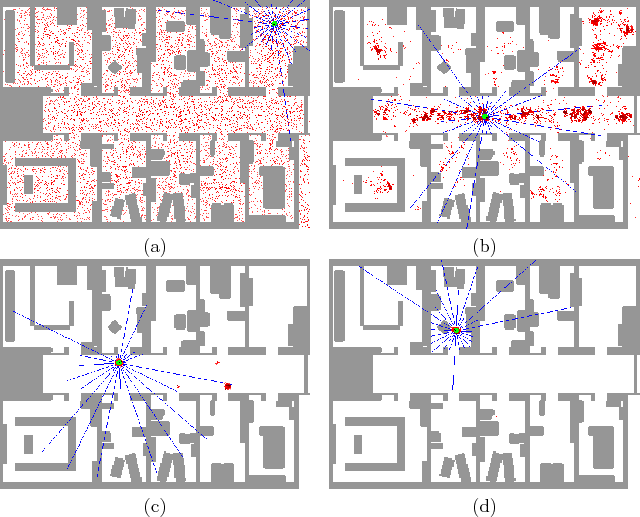Localization in a continuous space using probabilistic models has received substantial attention in recent years [258,447,622,825,887,962]. It is often difficult to localize mobile robots because of noisy sensor data, modeling errors, and high demands for robust operation over long time periods. Probabilistic modeling and the computation of probabilistic I-states have been quite successful in many experimental systems, both for indoor and outdoor mobile robots. Figure 12.11 shows localization successfully being solved using sonars only. The vast majority of work in this context involves passive localization because the robot is often completing some other task, such as reaching a particular part of the environment. Therefore, the focus is mainly on computing the probabilistic I-states, rather than performing a difficult search on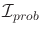.

Probabilistic localization in continuous spaces most often involves the definition of the probability densities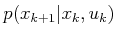and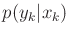(in the case of a state sensor mapping). If the stages represent equally spaced times, then these densities usually remain fixed for every stage. The state space is usually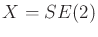to account for translation and rotation, but it may be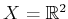for translation only. The densityaccounts for the unpredictability that arises when controlling a mobile robot over some fixed time interval. A method for estimating this distribution for nonholonomic robots by solving stochastic differential equations appears in .

The densityindicates the relative likelihood of various measurements when given the state. Most often this models distance measurements that are obtained from a laser range scanner, an array of sonars, or even infrared sensors. Suppose that a robot moves around in a 2D environment and takes depth measurements at various orientations. In the robot body frame, there areangles at which a depth measurement is taken. Ideally, the measurements should look like those in Figure 11.15b; however, in practice, the data contain substantial noise. The observation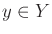is an-dimensional vector of noisy depth measurements.

One common way to define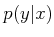is to assume that the error in each distance measurement follows a Gaussian density. The mean value of the measurement can easily be calculated as the true distance value onceis given, and the variance should be determined from experimental evaluation of the sensor. If it is assumed that the vector of measurements is modeled as a set of independent, identically distributed random variables, a simple product of Guassian densities is obtained for.

Once the models have been formulated, the computation of probabilistic I-states directly follows from Sections 11.2.3 and 11.4.1. The initial condition is a probability density function,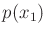, over. The marginalization and Bayesian update rules are then applied to construct a sequence of density functions of the form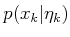for every stage,.

In some limited applications, the models used to expressandmay be linear and Gaussian. In this case, the Kalman filter of Section 11.6.1 can be easily applied. In most cases, however, the densities will not have this form. Moment-based approximations, as discussed in Section 11.4.3, can be used to approximate the densities. If second-order moments are used, then the so-called extended Kalman filter is obtained, in which the Kalman filter update rules can be applied to a linear-Gaussian approximation to the original problem. In recent years, one of the most widely accepted approaches in experimental mobile robotics is to use sampling-based techniques to directly compute and estimate the probabilistic I-states. The particle-filtering approach, described in general in Section 11.6.2, appears to provide good experimental performance when applied to localization. The application of particle filtering in this context is often referred to as Monte Carlo localization; see the references at the end of this chapter.

Steven M LaValle 2020-08-14